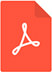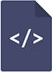# Parameter estimation for the bivariate inverse lomax distribution by the EM algorithm based on censored samplesPDF Full Text

# Abstract

In this article, we estimate the parameters of the bivariate Inverse Lomax distribution of Marshall-Olkin based on right censored sample. Utilizing EM algorithm is a priority because the vector of the observed data is not complete but viewed as an observable function of complete data. After that, the EM algorithm makes use of the simplicity of maximum likelihood estimation for complete data. In addition, normal deviations of the estimates of bivariate Lomax distribution are derived. A comparison is conducted via a simulation study between estimates obtained by using the EM algorithm and without the EM algorithm.

# Keywords

bivariate lomax distribution, censored sample, EM algorithm, missing data, simulation study